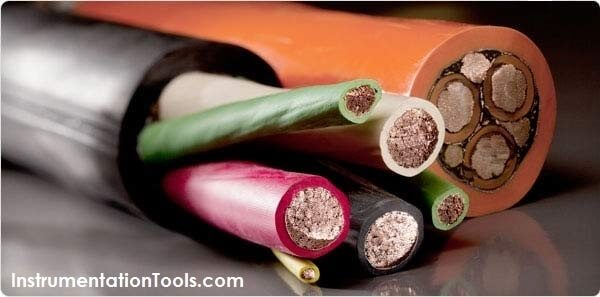# Basic Principles of ElectricityElectricity

Electricity, simply put, is the flow of electric current along a conductor. This electric current takes the form of free electrons that transfer from one atom to the next. Thus, the more free electrons a material has, the better it conducts. There are three primary electrical parameters: the volt, the ampere and the ohm.

1. The Volt

The pressure that is put on free electrons that causes them to flow is known as electromotive force (EMF). The volt is the unit of pressure, i.e., the volt is the amount of electromotive force required to push a current of one ampere through a conductor with a resistance of one ohm.

2. The Ampere

The ampere defines the flow rate of electric current. For instance, when one coulomb (or 6 x 1018 electrons) flows past a given point on a conductor in one second, it is defined as a current of one ampere.

3. The Ohm

The ohm is the unit of resistance in a conductor. Three things determine the amount of resistance in a conductor: its size, its material, e.g., copper or aluminum, and its temperature. A conductor’s resistance increases as its length increases or diameter decreases. The more conductive the materials used, the lower the conductor resistance becomes. Conversely, a rise in temperature will generally increase resistance in a conductor.

Ohm’s Law

Ohm’s Law defines the correlation between electric current (I), voltage (V), and resistance (R) in a conductor.

Ohm’s Law can be expressed as: V = I × R

Where: V = volts, I = amps, R = ohms

Ampacity

Ampacity is the amount of current a conductor can handle before its temperature exceeds accepted limits. These limits are given in the National Electrical Code (NEC), the Canadian Electrical Code and in other engineering documents such as those published by the Insulated Cable Engineers Association (ICEA). It is important to know that many external factors affect the ampacity of an electrical conductor and these factors should be taken into consideration before selecting the conductor size.